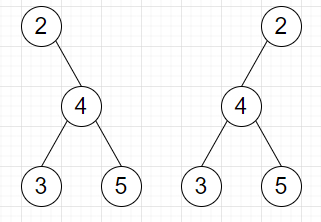# Program to check whether two trees can be formed by swapping nodes or not in Python

Suppose we have two trees, we have to check whether we can transform first tree into second one by swapping any node's left and right subtrees any number of times.

So, if the input is likethen the output will be True

To solve this, we will follow these steps −

• que1 := a queue with root0 initially

• que2 := a queue with root1 initially

• while que1 and que2 are not empty, do

• temp1 := a new list, temp2 := a new list

• values1 := a new list, values2 := a new list

• if que1 and que2 are containing different number of elements, then

• return False

• for i in range 0 to size of que1 − 1, do

• insert value of que1[i] at the end of values1

• insert value of que2[i] at the end of values2

• if right of que1[i] is not null, then

• insert right of que1[i] at the end of temp1

• if left of que1[i] is not null, then

• insert left of que1[i] at the end of temp1

• if right of que2[i] is not null, then

• insert right of que2[i] at the end of temp2

• if left of que2[i] is not null, then

• insert left of que2[i] at the end of temp2

• if values1 is not same as values2, then

• if values1 is not same as values2 in reverse order, then

• return False

• que1 := temp1, que2 := temp2

• return True

Let us see the following implementation to get better understanding −

## Example

Live Demo

class TreeNode:
def __init__(self, data, left = None, right = None):
self.val = data
self.left = left
self.right = right
class Solution:
def solve(self, root0, root1):
que1 = [root0]
que2 = [root1]
while que1 and que2:
temp1 = []
temp2 = []
values1 = []
values2 = []
if len(que1) != len(que2):
return False
for i in range(len(que1)):
values1.append(que1[i].val)
values2.append(que2[i].val)
if que1[i].right:
temp1.append(que1[i].right)
if que1[i].left:
temp1.append(que1[i].left)
if que2[i].right:
temp2.append(que2[i].right)
if que2[i].left:
temp2.append(que2[i].left)
if values1 != values2:
if values1 != values2[::-1]:
return False
que1 = temp1
que2 = temp2
return True
ob = Solution()
root = TreeNode(2)
root.right = TreeNode(4)
root.right.left = TreeNode(3)
root.right.right = TreeNode(5)
root1 = TreeNode(2)
root1.left = TreeNode(4)
root1.left.left = TreeNode(3)
root1.left.right = TreeNode(5)
print(ob.solve(root, root1))

## Input

root = TreeNode(2)
root.right = TreeNode(4)
root.right.left = TreeNode(3)
root.right.right = TreeNode(5)
root1 = TreeNode(2)
root1.left = TreeNode(4)
root1.left.left = TreeNode(3)
root1.left.right = TreeNode(5)

## Output

True